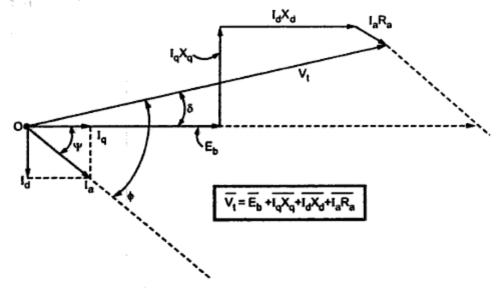### Salient Pole Synchronous Motor

The analysis of salient pole synchronous motor is based on the Blondel's two reaction. The direct and quadrature axis components of current and reactance are same as defined earlier for the synchronous generators. Thus,
Xd = Direct axis reactance
I= Direct axis component of Ia
Iq = Quadrature axis component of Ia
The complete phasor diagram of lagging p.f. is shown in the Fig. 1.Fig. 1 Phasor diagram for lagging p.f.
From the phasor diagram it can be derived that,
Note : For the proof of above results refer example 2.
The complete phasor diagram of leading p.f. is shown in the Fig. 2.Fig. 2  Phasor diagram for leading p.f.